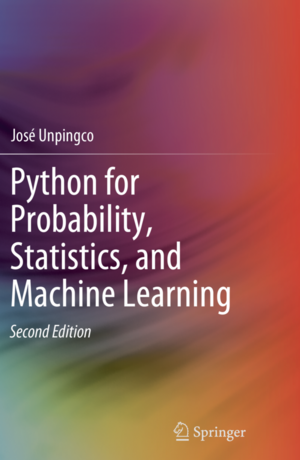# Python For Probability Statistics And Machine Learning 2e

Second edition of Springer Book Python for Probability, Statistics, and Machine Learning
Alternatives To Python For Probability Statistics And Machine Learning 2e
Probability3,9171262135 days ago43June 07, 2022634apache-2.0Jupyter Notebook
Probabilistic reasoning and statistical analysis in TensorFlow
Math Php2,222362218 days ago132April 10, 202251mitPHP
Powerful modern math library for PHP: Features descriptive statistics and regressions; Continuous and discrete probability distributions; Linear algebra with matrices and vectors, Numerical analysis; special mathematical functions; Algebra
Stat Cookbook2,102
4 months ago2otherTeX
:orange_book: The probability and statistics cookbook
Www.mlcompendium.com1,991
12 days ago
The Machine Learning & Deep Learning Compendium was a list of references in my private & single document, which I curated in order to expand my knowledge, it is now an open knowledge-sharing project compiled using Gitbook.
Ml Foundations1,705
7 months agomitJupyter Notebook
Machine Learning Foundations: Linear Algebra, Calculus, Statistics & Computer Science
12 days ago1mit
Data Science Roadmap from A to Z
Hackermath1,339
6 years ago5mitJupyter Notebook
Introduction to Statistics and Basics of Mathematics for Data Science - The Hacker's Way
Seeing Theory1,043
4 years ago22apache-2.0HTML
A visual introduction to probability and statistics.
Distributions.jl992
7669 days agoJanuary 02, 2021361otherJulia
A Julia package for probability distributions and associated functions.
Kotlin Statistics82543a year ago7December 27, 201815apache-2.0Kotlin
Idiomatic statistical operators for Kotlin
Alternatives To Python For Probability Statistics And Machine Learning 2e
Select To Compare

Alternative Project Comparisons

# Python-for-Probability-Statistics-and-Machine-Learning-2EThis book, fully updated for Python version 3.6+, covers the key ideas that link probability, statistics, and machine learning illustrated using Python modules in these areas. All the figures and numerical results are reproducible using the Python codes provided. The author develops key intuitions in machine learning by working meaningful examples using multiple analytical methods and Python codes, thereby connecting theoretical concepts to concrete implementations. Detailed proofs for certain important results are also provided. Modern Python modules like Pandas, Sympy, Scikit-learn, Tensorflow, and Keras are applied to simulate and visualize important machine learning concepts like the bias/variance trade-off, cross-validation, and regularization. Many abstract mathematical ideas, such as convergence in probability theory, are developed and illustrated with numerical examples.

This updated edition now includes the Fisher Exact Test and the Mann-Whitney-Wilcoxon Test. A new section on survival analysis has been included as well as substantial development of Generalized Linear Models. The new deep learning section for image processing includes an in-depth discussion of gradient descent methods that underpin all deep learning algorithms. As with the prior edition, there are new and updated Programming Tips that the illustrate effective Python modules and methods for scientific programming and machine learning. There are 445 run-able code blocks with corresponding outputs that have been tested for accuracy. Over 158 graphical visualizations (almost all generated using Python) illustrate the concepts that are developed both in code and in mathematics. We also discuss and use key Python modules such as Numpy, Scikit-learn, Sympy, Scipy, Lifelines, CvxPy, Theano, Matplotlib, Pandas, Tensorflow, Statsmodels, and Keras.

This book is suitable for anyone with an undergraduate-level exposure to probability, statistics, or machine learning and with rudimentary knowledge of Python programming.

## Conda setup instructions

If you are using `conda`, you can get started by cloning this repository and using the `environment.yaml` file as in the following:

``````conda env create -n pyPSML -f environment.yaml
``````

and then activate the environment using the following,

``````conda activate pyPSML
``````

Then, you can run `jupyter notebook` and navigate the Jupyter notebooks for the individual chapters. All of the notebooks are fully functional in this so-created environment. Note that there are embedded figures in the Jupyter notebooks that are meant to validate the outputs of the Matplotlib codes therein.

## Docker setup instructions

If you are using docker, there is a `Dockerfile` included. After cloning this repository, you can build the image with the following,

``````docker build -t pypsml2e .
``````

and then run it locally using,

``````docker container run -it -p 8888:8888 pypsml2e
``````

Then, navigate to the output URL and you can explore the Jupyter notebooks for each chapter. Alternately, if you don't want to build your own image, you can do

``````docker run -p 8888:8888 unpingco/pypsml2e
``````

to get the docker image from https://hub.docker.com/r/unpingco/pypsml2e. Note that this may not be as up-to-date as building it yourself from this repository, but should still work fine.

Good luck! I hope you find these materials helpful.

Popular Statistics Projects
Popular Probability Projects
Popular Data Processing Categories
Related Searches

Get A Weekly Email With Trending Projects For These Categories
No Spam. Unsubscribe easily at any time.
Python
Jupyter Notebook
Machine Learning
Statistics
Probability